• 用python的matplotlib画图时，往往需要加图例说明。如果不设置任何参数，默认是加到图像的内侧的最佳位置。...这样的结果如所示： 如果需要将该legend移到图像外侧，有多种方法，这里介绍一种。
• 【Python】Matplotlib切割图片

千次阅读 2020-02-13 14:37:55
Matplotlib可以切割图片。这里用圆形切了原图片，并隐去了坐标轴，只关注图案本身，来看~

图像的处理

从外部导入的图像通常是以图片的形式存在的，图片外观样式一般是矩形。

如果需要将矩形图片以其他样式在坐标轴上进行展示，那么这个需求就需要借助图片剪切、加载和展示等方法加以实现。

这里用圆形切了原图片，并隐去了坐标轴，只关注图案本身，来看~

原图Matplotlib编程实现

import matplotlib.pyplot as plt
from matplotlib.cbook import get_sample_data
from matplotlib.patches import Circle

with get_sample_data("d:\PyCharm\data\pig.jpg", asfileobj=True) as imageFile:

fig, ax = plt.subplots(1, 1)
ai = ax.imshow(imageArray)
patch = Circle((125, 125), radius=125, transform=ax.transData)
ai.set_clip_path(patch)

ax.set_axis_off()

plt.show()

成品图展开全文• 最近学了matplotlib，想要在绘制3D图像的时候插入图片，但在网上找来找去没找到比较好的，唯一找到的一个速度有点慢。于是就自己动手吧。 先放下在stackoverflow看到的答案： # 注释为国外大佬写的，汉字部分我的...

最近学了matplotlib，想要在绘制3D图像的时候插入图片，但在网上找来找去没找到比较好的，唯一找到的一个速度有点慢。于是就自己动手吧。

先放下在stackoverflow看到的答案：

# 注释为国外大佬写的，汉字部分我的补充
import numpy as np
import matplotlib.pyplot as plt
from mpl_toolkits.mplot3d import Axes3D
from matplotlib.cbook import get_sample_data
import cv2

# Read the image with Opencv
# Change the color from BGR to RGB
img = cv2.cvtColor(img, cv2.COLOR_BGR2RGB)

# Orgird to store data
x, y = np.ogrid[0:img.shape, 0:img.shape]
# In Python3 matplotlib assumes rgbdata in range 0.0 to 1.0
img = img.astype('float32')/255
fig = plt.Figure()
# gca do not work thus use figure objects inbuilt function.

# Plot data
# rstride，cstride相当于设置图片显示的像素，当两项均等于1的时候相当于我写的程序
ax.plot_surface(x, y, np.atleast_2d(0), rstride=10, cstride=10, facecolors=img)
fig.show() # Throws a AttributeError

这个方法我试了试，但有点慢，画一个800*800的图片需要将近50s。

matplotlib的3D的画图应该是没有直接提供插入图片的选项的，那自己划入图片的思维最简单就是一个一个的写入。

使用Image先获得图片，再读取其size，其中size为宽，size为高。

img = Image.open('african.jpg')
width = img.size
height = img.size

获得宽高之后便可以使用numpy创建x,y轴的列表。我的图片是800*800的，所以这里的宽高就是两个长度为800的列表。

而后使用meshgrid()函数（实际上我并不清楚这个函数的用处，应该是类似将两个数组中的元素匹配。如果没有这一步，画出来的图将会只有800个点，有了这一步才能画出一张640000像素的画。如果有大佬知道这个函数具体干啥的希望能指导下）

接下来创建图像的z轴坐标。因为这张图像使用的是散点画法，总共要画640000个点，所以应该有这么多的z轴坐标，并且应该将其设置为一个一维数组而不是二维，二维数组放入应该会报错。

x = np.arange(0,width)
y = np.arange(0,height)
x,y = np.meshgrid(x,y)
z = np.zeros(width*height)

然后再处理颜色。根据其官方文档中的关于color的描述，可以看出c传入的参数可以是一个代表颜色的字符串，或者是一个长度为N的列表，或者是一个2维的数组。但二维数组我尝试过却报错了，不知道是我哪里写错了。有大佬的画希望可以指点一下。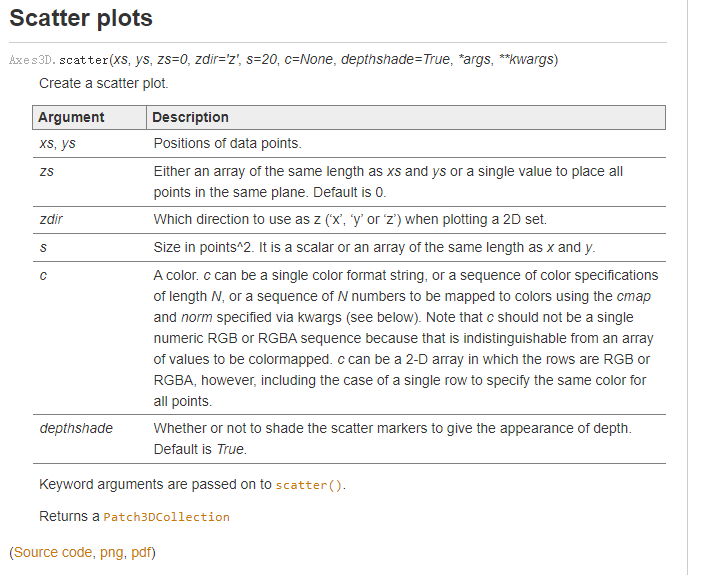那么剩下的就是获取每个像素点的像素值了。通过遍历长宽可以获得每个像素点的rgb值，其中pix[x,y]返回的按次序为rgb的十进制值。但color参数似乎并不支持直接传10进制的rgb值，他支持16进制的颜色值或者是一个三元素元组，其中每个元素代表这种颜色所占比例，因此每个10进制的值需要除以255。

如果不需要每个像素都一样的，可以设置其步长，效果相当于前面代码的更改rstride的值。

color = []
for i in range(width):
for j in range(height):
rgb= tuple(np.array(pix[i, j])/255)
color.append(rgb)

最后直接开始画图。alpha = 1代表不透明度为100%

ax.scatter(x,y,z,c = color,alpha = 1)

运行结果如下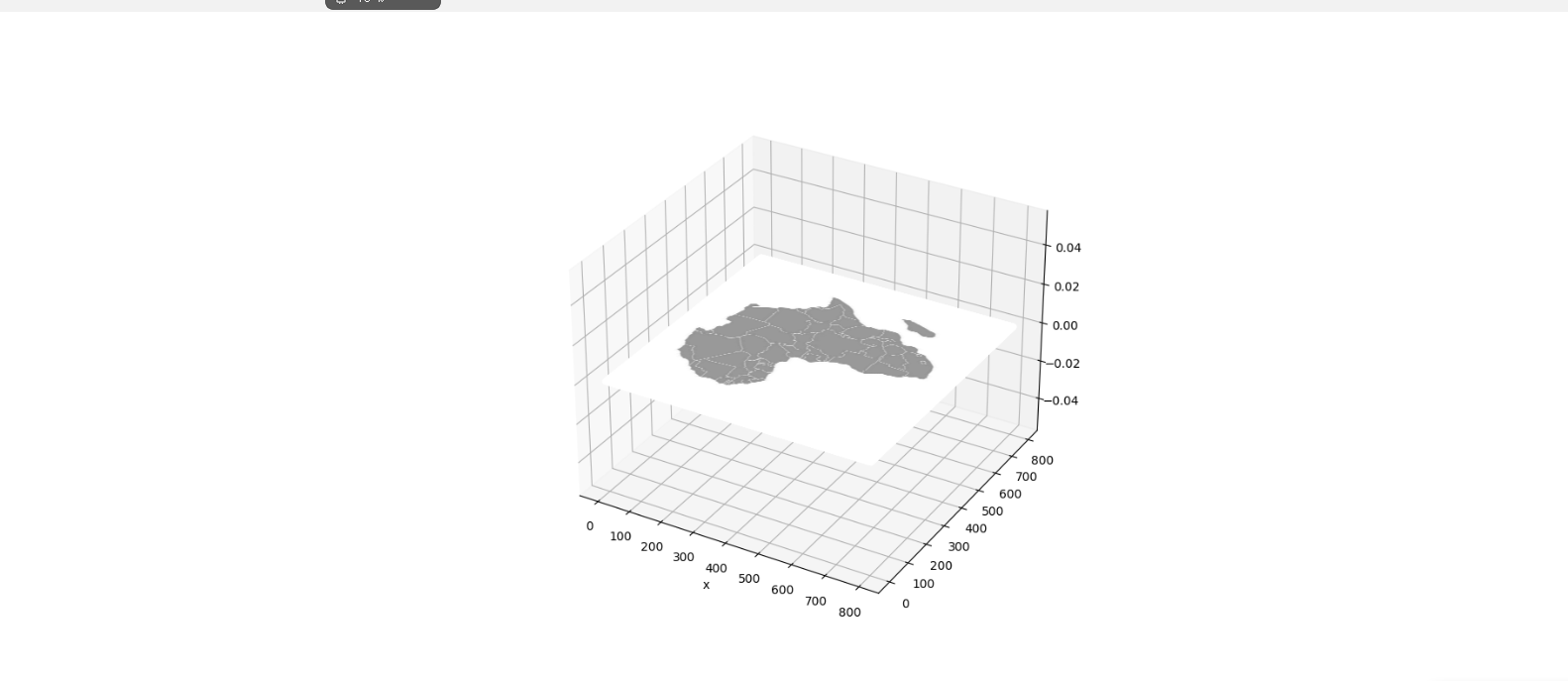下面是完整的代码，可以和上面的代码实现一样的功能，速度更快了，运行大概需要15s：

from PIL import Image
import numpy as np
from matplotlib import pyplot as plt

# 创建3D对象
fig = plt.figure(figsize=(20,8),dpi=100)

# 读取图片
img = Image.open('test.jpg')
# 获取图片长宽
width = img.size
height = img.size
# 创建x,y轴的长度
x = np.arange(0,width)
y = np.arange(0,height)
x,y = np.meshgrid(x,y)
z = np.zeros(width*height)
# 建立列表，后期使用
color = []
# 遍历长宽，获得每个像素点的RGB值
for i in range(width):
for j in range(height):
# 转化rgb为相对占比的元组
rgb= tuple(np.array(pix[i, j])/255)
color.append(rgb)

# 在z=0的平面上作图

# 设置颜色，像素
ax.scatter(x,y,z,c = color,alpha = 1)
plt.show()

代码可以再优化，比如连续出现的相同像素等等。

新手第一次发帖，纯萌新，有错误希望大佬指出。

展开全文python matplotlib
• 需要对像素矩阵转换成图片进行保存，暂且发现可通过matplotlib来实现，但默认生成的图片有白框，故寻找了一种通过子图避免白框的方法。 # 图片分辨率 = figsize*dpi 代码为512*512 plt.rcParams['figure.figsize']...

问题描述

在使用Matplotlib对图片而不是图表进行操作时，常需要存储无坐标轴和白边框的图片。

解决方法

需要对像素矩阵转换成图片进行保存，暂且发现可通过matplotlib来实现，通过子图，可避免出现白边框。

# 图片分辨率 = figsize*dpi 代码为512*512
plt.rcParams['figure.figsize'] = (10.24, 10.24)
plt.rcParams['savefig.dpi'] = 50
# 去除白框
plt.axis('off')
plt.margins(0,0)
# 保存图片，cmap为调整配色方案
plt.imshow(img_arr,cmap=plt.cm.gray)
plt.savefig(filename + ".jpg")

展开全文python matplotlib 机器学习 深度学习
• python matplotlib如何将图例放在图外

千次阅读 多人点赞 2017-03-13 17:14:05
关于matplotlib如何设置图例的位置...如何将图例放在图外？以及如何在一幅有多个子图的情况下，删除重复的图例？我用一个简单的例子说明一下。 import pandas as pd import numpy as np import matplotlib.pyplot

原文地址：http://blog.csdn.net/john_xyz/article/details/54754937

关于matplotlib如何设置图例的位置？如何将图例放在图外？以及如何在一幅图有多个子图的情况下，删除重复的图例？我用一个简单的例子说明一下。

import pandas as pd
import numpy as np
import matplotlib.pyplot as plt

fig = plt.figure(1)

df1 = pd.DataFrame(np.random.randn(3,5),columns = ['one','two','three','four','five'])
df2 = pd.DataFrame(np.random.randn(3,5),columns = ['one','two','three','four','five'])
df3 = pd.DataFrame(np.random.randn(3,5),columns = ['one','two','three','four','five'])
df4 = pd.DataFrame(np.random.randn(3,5),columns = ['one','two','three','four','five'])

df1.plot(ax = ax1, title = "df1", grid = 'on')
df2.plot(ax = ax2, title = "df1", grid = 'on')
df3.plot(ax = ax3, title = "df1", grid = 'on')
df4.plot(ax = ax4, title = "df1", grid = 'on')

plt.show()

• 1
• 2
• 3
• 4
• 5
• 6
• 7
• 8
• 9
• 10
• 11
• 12
• 13
• 14
• 15
• 16
• 17
• 18
• 19
• 20
• 21
• 22
• 23
• 1
• 2
• 3
• 4
• 5
• 6
• 7
• 8
• 9
• 10
• 11
• 12
• 13
• 14
• 15
• 16
• 17
• 18
• 19
• 20
• 21
• 22
• 23

运行结果如下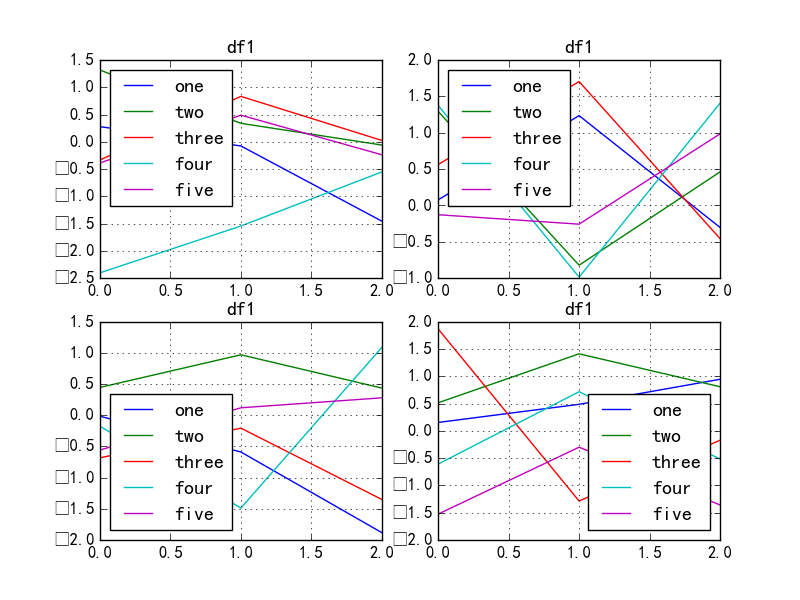可以看出，随机生成了几个dataframe,在一个figure()中生成了四个子图，每个子图的图例都是dataframe.columns里的值，那么如何移除这些图例？

import pandas as pd
import numpy as np
import matplotlib.pyplot as plt

fig = plt.figure(1)

df1 = pd.DataFrame(np.random.randn(3,5),columns = ['one','two','three','four','five'])
df2 = pd.DataFrame(np.random.randn(3,5),columns = ['one','two','three','four','five'])
df3 = pd.DataFrame(np.random.randn(3,5),columns = ['one','two','three','four','five'])
df4 = pd.DataFrame(np.random.randn(3,5),columns = ['one','two','three','four','five'])

df1.plot(ax = ax1, title = "df1", grid = 'on')
df2.plot(ax = ax2, title = "df1", grid = 'on')
df3.plot(ax = ax3, title = "df1", grid = 'on')
df4.plot(ax = ax4, title = "df1", grid = 'on')

ax1.legend_.remove()        ##移除子图ax1中的图例
ax2.legend_.remove()        ##移除子图ax2中的图例
ax3.legend_.remove()        ##移除子图ax3中的图例

plt.show()

• 1
• 2
• 3
• 4
• 5
• 6
• 7
• 8
• 9
• 10
• 11
• 12
• 13
• 14
• 15
• 16
• 17
• 18
• 19
• 20
• 21
• 22
• 23
• 24
• 25
• 26
• 27
• 28
• 1
• 2
• 3
• 4
• 5
• 6
• 7
• 8
• 9
• 10
• 11
• 12
• 13
• 14
• 15
• 16
• 17
• 18
• 19
• 20
• 21
• 22
• 23
• 24
• 25
• 26
• 27
• 28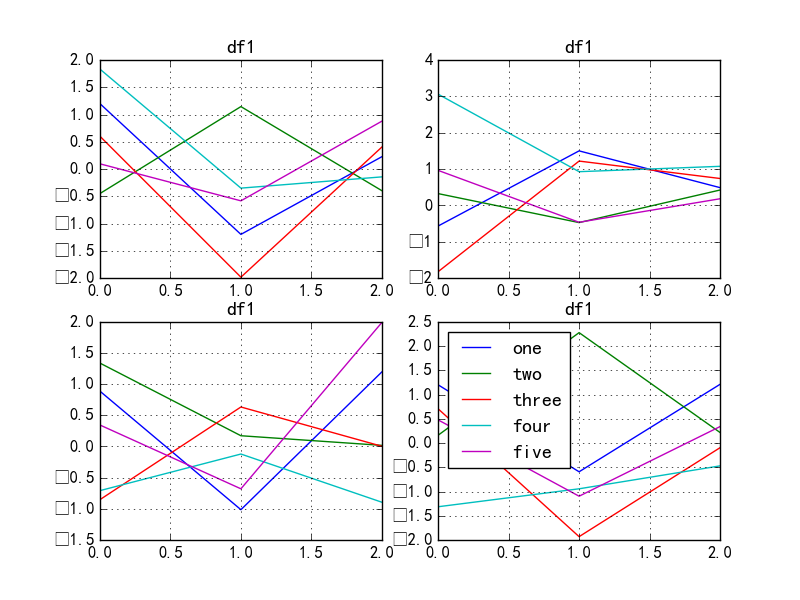可以看出ax1,ax2,ax3中的图例都被移除了，但是上图还不是很美观？有没有什么办法将图例放到图外面呢？请看:

import pandas as pd
import numpy as np
import matplotlib.pyplot as plt

fig = plt.figure(1)

df1 = pd.DataFrame(np.random.randn(3,5),columns = ['one','two','three','four','five'])
df2 = pd.DataFrame(np.random.randn(3,5),columns = ['one','two','three','four','five'])
df3 = pd.DataFrame(np.random.randn(3,5),columns = ['one','two','three','four','five'])
df4 = pd.DataFrame(np.random.randn(3,5),columns = ['one','two','three','four','five'])

df1.plot(ax = ax1, title = "df1", grid = 'on')
df2.plot(ax = ax2, title = "df1", grid = 'on')
df3.plot(ax = ax3, title = "df1", grid = 'on')
df4.plot(ax = ax4, title = "df1", grid = 'on')

ax1.legend_.remove()
ax2.legend_.remove()
ax3.legend_.remove()

plt.show()

• 1
• 2
• 3
• 4
• 5
• 6
• 7
• 8
• 9
• 10
• 11
• 12
• 13
• 14
• 15
• 16
• 17
• 18
• 19
• 20
• 21
• 22
• 23
• 24
• 25
• 26
• 27
• 28
• 1
• 2
• 3
• 4
• 5
• 6
• 7
• 8
• 9
• 10
• 11
• 12
• 13
• 14
• 15
• 16
• 17
• 18
• 19
• 20
• 21
• 22
• 23
• 24
• 25
• 26
• 27
• 28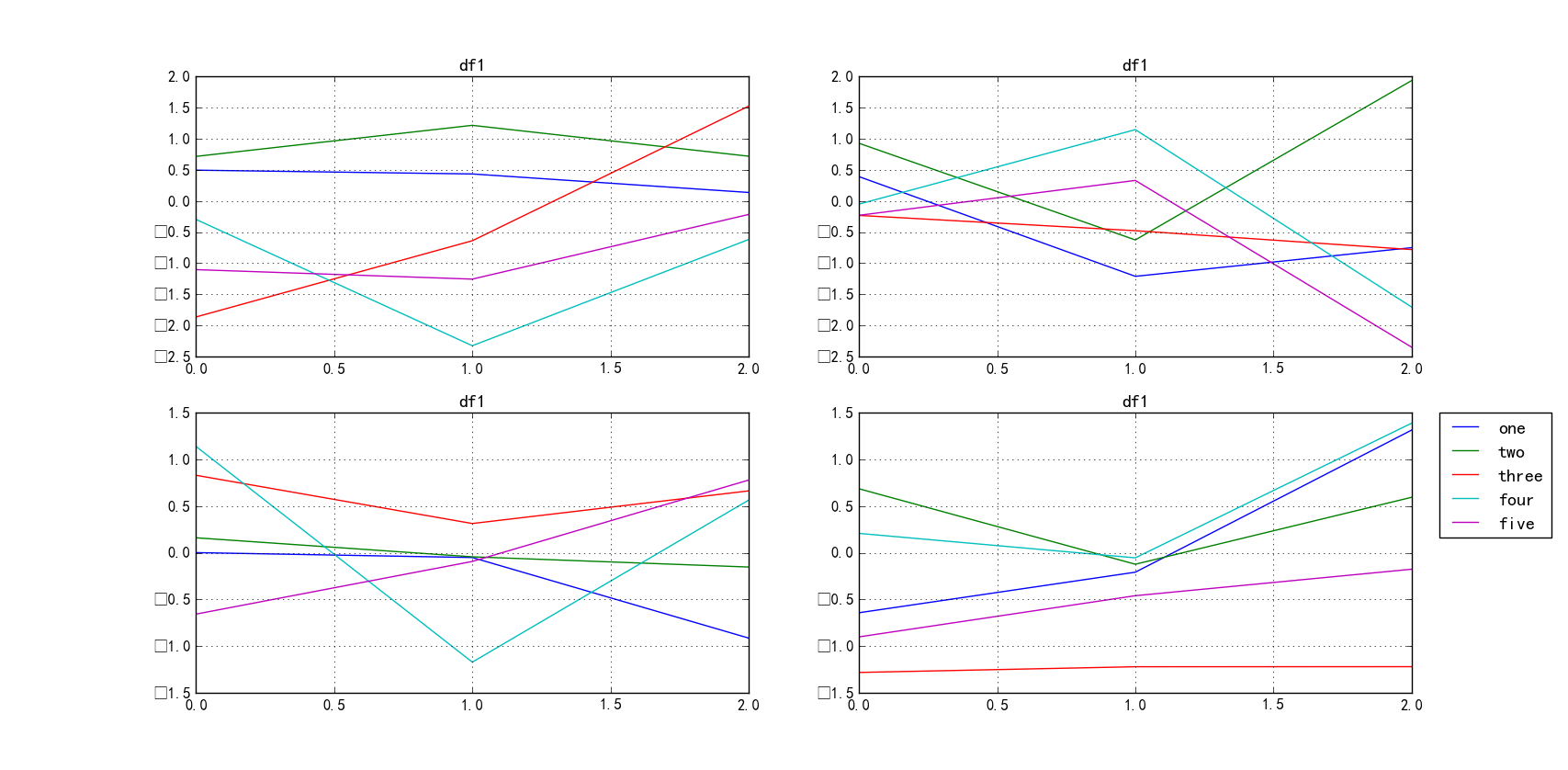其中参数loc用于设置legend的位置
bbox_to_anchor用于在bbox_transform坐标（默认轴坐标）中为图例指定任意位置。

关于plt.legend更多的参数信息，详细见官方文档：http://matplotlib.org/api/pyplot_api.html#matplotlib.pyplot.legend
关于legend的官方教程：详细见：http://matplotlib.org/users/legend_guide.html
关于pandas.DataFrame.plot更多的作图问题，详细见：http://pandas.pydata.org/pandas-docs/stable/generated/pandas.DataFrame.plot.html

展开全文• 点击上方“算法猿的成长“，关注公众号，选择加“星标“或“置顶”总第130 篇文章，本文大约1000字，阅读大约需要5 分钟前言今天简单介绍如何通过 matplotlib 展示图片...
• import matplotlib as mpl import matplotlib.pyplot as plt import seaborn as sns 数据源： data = pd.DataFrame( data=[[1,2.1],[2,3.2],[3,4.3],[4,5.4]],columns=['x','y']) 内容： x y 0 1 2.1 1 2 3.2 2 ...matplotlib
• Matplotlib具有广泛的文本支持，包括对数学表达式的支持、对栅格和矢量输出的TrueType支持、具有任意旋转的换行分隔文本以及Unicode支持。 下面的命令是介绍了通过pyplot API和objected-oriented API分别创建文本的...
• Python matplotlib绘制折线图

千次阅读 2020-11-01 22:10:31matplotlib 折线图
• Matplotlib三层结构Matplotlib实操之折线(plot)与基础绘图功能折线绘制与保存图片实际应用之显示图片实际应用之保存图片实际应用之完善原始折线1(辅助显示层) Matplotlib介绍 什么是Matplotlib 是专门用于...python jupyter matplotlib
• matplotlib

千次阅读 多人点赞 2019-04-20 19:19:46
今天我们来介绍一下python的一个可视化工具matplotlib matplotlib 使用matplotlib的方式有很多，但最通常的是Pylab模式的ipython(-ipython –pylab) matplotlib的api都位于matplotlib.pyplot中，所以一般的引入方式...matplotlib
• import matplotlib.pyplot as plt x = [1,2,3,4,5] y = [1,2,3,4,5] plt.plot(x,y) plt.show() 代码运行结果如下： 二、如何添加图例？ 注意在使用matplotlib画图的时候最好加上这几行代码解决中文显示问题 from ...python 开发语言 后端
• fig, ax = plt.subplots() ax.一系列绘图操作（） plt.axis("off") # 去除图像周围的白边 plt.gca().xaxis.set_major_locator(plt.NullLocator()) plt.gca().yaxis.set_major_locator(plt.NullLocator()) ...python matplotlib
• Python matplotlib绘制雷达图

千次阅读 2020-12-20 23:09:48
• bar()函数用于绘制柱形。 bar()的函数签名为matplotlib.pyplot.bar(x, height, width=0.8, bottom=None, *, align='center', data=None, **kwargs)。 bar()的返回值为BarContainer对象，其中patche属性为Rectangle...matplotlib pyplot bar 柱状图
• 本文主要介绍基于matplotlib实现圆环。本文所有代码见：Python-Study-Notes # 去掉警告 import warnings warnings.filterwarnings("ignore") # 多行输出 from IPython.core.interactiveshell import Interactive...
• 6. 直方和密度：hist() 7. 多合并显示：subplot()和subplots() 8. 图表动态刷新：ion()和ioff() 线图：plot() 函数功能：展现变量的变化趋势 调用方法：plt.plot(x, y, linestyle, linewidth,color,marker, ...数据可视化
• 保存了图片之后，保存的图片不存在横纵坐标轴 保存图片代码 plt.savefig('../../Data/Pics/MeanFig.png', dpi = 200, bbox_inches = 'tight') 代码运行结果 运行时结果 保存图片结果 解决办法 把图片格式后缀...python matplotlib
• Matplotlib三维绘图，这一篇就够了。这篇博客将介绍3D线图、散点、随机颜色散点、散点不同mark点图、线框、曲面不透明、曲面透明等7种3D效果python 数据可视化
• 调整折线：线条颜色和风格 你可能第一个想到需要进行调整的部分就是线条的颜色和风格。plt.plot()函数接受额外的参数可以用来指定它们。通过指定color关键字参数可以调整颜色，这个字符串类型参数基本上能用来代表...python matplotlib
• 在用Matplotlib进行可视化过程中，很多时候为了更直观地展现数据大小，会将具体的数值标注在图形中，比如在柱状上标明数值大小。这篇文章会以一个实际的案例，详细讲讲如何给数据加注解，...
• 先看一下原来正常的时候是什么样子的，在jupyterlab中，这个图片只能通过代码缩放 基至是保存， 若想保存这张图，也只能通过右键创建新视图后才能保存下来 那么问题来了，我们想要和这张图片交互，应该怎么办呢 1....python matplotlib
• from matplotlib import pyplot as plt x = range(1,10) #x轴的位置 y = [6,7,12,12,15,17,15,20,18] #y轴的位置 #传入x,y，通过plot画图,并设置折线颜色、透明度、折线样式和折线宽度 标记点、标记点大小、标记点边...matplotlib
• grid()的函数签名为matplotlib.pyplot.grid(b=None, which='major', axis='both', **kwargs)。 grid()的参数如下： b：是否显示网格线。布尔值或None，可选参数。如果没有关键字参数，则b为True，如果b为None且没有...matplotlib pyplot grid 网格线 外观
• matplotlib绘图样式(style)初探

万次阅读 2021-01-03 10:20:45
Styles are predefined sets ...Customizing Matplotlib with style sheets and rcParams describes the mechanism and usage of styles. The Style sheets reference gives an overview of the builtin styles. matpl
•python matplotlib 数据分析
• 如果两个df大小不一样，选错内、连接会丢失数据 df3=pd.merge(df1_result,df2_result,on='购买日期') #**************************************************************** #(2)将（1）的结果绘图 fig,axs=plt....
• 同时，可以使用这些工具创建各种图形：包括简单的散点、正弦曲线，甚至是三维图形； 在 Python 科学计算社区，经常使用它完成数据可视化的工作；在接下来的梳理，学习一下这个库的神奇功能！ 如果使用的是Anaconda...python matplotlib 数据可视化 实时数据
• import matplotlib.pyplot as plt plt.figure(figsize=(9,9)) # 环形饼图元素 recipe = ["225g flour", "90g sugar", "1 egg", "60g butter", "100ml milk", "1/2package of yeast"] # ⽐例 data = [225, 90python 数据可视化 matplotlib
• 我们学习了使用 OpenCV 提供的 cv2.calcHist() 函数来计算直方。...出于提高性能目的，我们来比较这些函数，使用 OpenCV、NumPy 和 Matplotlib 创建直方，然后测量每个直方计算的执行时间并将结果绘制在图形中。原力计划 python opencv 人工智能 直方图...